Courses

# Motion under gravity Class 11 Notes | EduRev

## NEET : Motion under gravity Class 11 Notes | EduRev

The document Motion under gravity Class 11 Notes | EduRev is a part of the NEET Course Physics For JEE.
All you need of NEET at this link: NEET

2. Motion under gravity :

I format : (When a body is thrown vertically upward)

It includes two types of motion
(i) Deaccelerated motion from A to B because the direction of velocity and acceleration is opposite. So speed
decreases
(ii) Accelerated motion from B to C because the direction of velocity and acceleration is same (downward). So speed increases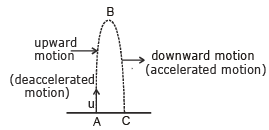(a) Time of flight :

It is the time taken by the particle to reach the ground. If the particle is thrown vertically upward with initial velocity u then
u= u
a = – g (take downward direction negative)
from equation
S = ut +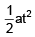⇒  Snet = 0 (when particle again reaches the ground)
t = T (time of flight)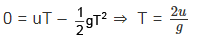(b) Maximum Height :

from v2 = u2 + 2as

at maximum height v = 0, s = Hmax
⇒  0 = u2 –2 gHmax   ⇒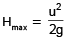(c) Final velocity

from v = u + at
v = vf a = – g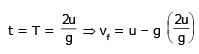v= – u
i.e. the body reaches the ground with the same speed with which it was thrown vertically upwards as it thrown vertically upward.

(d) Time to reach half of the maximum height :-

ui = u   a = – g
from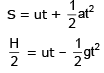H = 2ut – gt2   ⇒  gt– 2ut + H = 0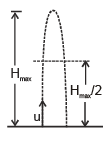⇒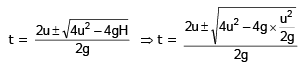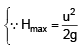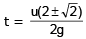(.....1)

Equation 1 gives two value of time which corresponds to
t1 =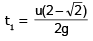(from ground to Hmax/2 in upward motion)
t2 =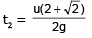(from  ground to Hmax/2 in downward motion)

(e) Time to reach any general height h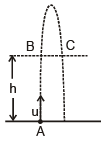Let us assume that particle reaches from A to B in time t&  from A to C is time t2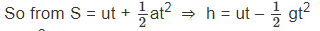gt2 – 2ut + 2h = 0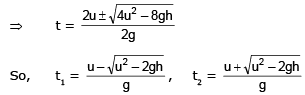⇒   t1  + t2 = T (Time of flight)

II Format (Free fall) :

A body released near the surface of the earth is accelerated downward under the influence of force of gravity.

(a) Time of Flight :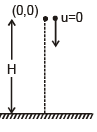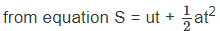S = – H, u = 0, a = – g
t = T (Let assume)
⇒ – H = (0)T –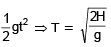(b) Final Velocity when body reaches the ground

from v2 – u2 = 2as
s = – H   v = v u = 0 a = – g
⇒   – 0  = 2 (–g) (–H)  ⇒  vf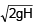Ex.17 A ball is thrown vertically upwards with a velocity u from the ground. The ball attains a maximum height Hmax. Then find out the time and displacement at which ball have half of the maximum speed.

Sol. Maximum speed of the ball is u  At point B and C ball have speed u/2 but direction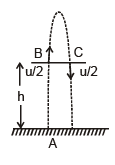is opposite so from
v = u + at
Let t1 is the time taken by the ball from point A to B and t2 is  the time taken by the ball from A to C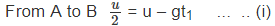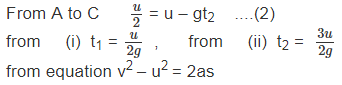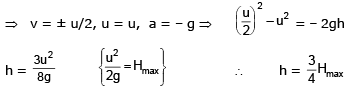Ex.18 A ball thrown vertically upwards with a speed of 19.6 ms–1 from the top of a tower returns to the earth in 6 s. Find the height of the tower.

Sol. Here u = 19.6 ms–1
g = –9.8 ms–2
Net displacement, s = – h
Negative sign is taken because displacement is in  the opposite direction of initial velocity.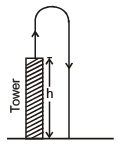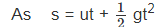∴  – h = 19.6 × 6 + 1/2 × (–9.8) × 62
= 117.6 – 176.4 = –58.8
or h = 58.8 m

Ex.19 A ball is thrown vertically upwards with a velocity of 20 ms–1 from the top of a multi-storeyed building. The height of the point from where the ball is thrown is 25 m from the ground. (i) How high will the ball rise and (ii) how long will it be before the ball hits the ground?

Sol. (i) Here u = +20 ms–1, g = –10 ms–2
At the highest point, v = 0
Suppose the ball rises to the height h from the point of projection.
As v2 – u2 = 2gs
∴   02 – 202 = 2 × (–10) × h    or h = + 20 m.
(ii) Net displacement, s = –25 m
Negative sign is taken because displacement is in the opposite direction of initial velocity.
As s = ut +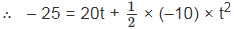or 5t2 – 20t – 25 = 0 or t2 – 4t – 5 = 0
or (t+ 1) (t – 5) = 0
As t ≠ –1,  so t = 5s.

Ex.20 A ball thrown up is caught by the thrower after 4s. How high did it go and with what velocity was it thrown ? How far was it below the highest point 3 s after it was thrown?

Sol. As time of ascent = time of descent
∴ Time taken by the ball to reach the highest point = 2 s
For upward motion of the ball : u = ?,  v = 0, t = 2s, g = – 9.8 ms–2
As v = u + gt
∴  0 = u – 9.8 × 2
or u = 19.6 ms–1
Maximum height attained by the ball is given by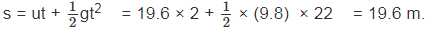Displacement of the ball in 3 s,
s = 19.6 × 3 +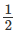× (–9.8) × 32     = 58.8 – 44.1 = 14.7 m
Distance of the ball from the highest point 3 s after it was thrown
= 19.6 – 14.7 = 4.9 m.

Ex.21 A balloon is ascending at the rate of 9.8 ms–1 at a height of 39.2 m above the ground when a food packet is dropped from the balloon. After how much time and with what velocity does it reach the ground?
Take g = 9.8 ms–2

Sol. Initially the food packet attains the upward velocity of the balloon, so
u = 9.8 ms–1, g = 9.8 ms–2 , s = –39.2 m
Here s is taken negative because it is in the opposite direction of initial velocity.
Using, s = ut + 1/2 gt2 , we get
– 39.2 = 9.8 t – 1/2× 9.8 t2
or  4.9 t2 – 9.8 t – 39.2 = 0    or t2 – 2t – 8 =0
or (t – 4) (t + 2) = 0      or t = 4s or – 2s
As time is never negative, so t = 4s.

Velocity with which the food packet reaches the ground is
v = u + gt = 9.8 – 9.8 × 4 = – 29.4 ms–1.
Negative sign shows that the velocity is directed vertically downwards.
When a particle is dropped then it will automatically attains the velocity of the frame at that time.

Ex.22 Two balls are thrown simultaneously, A vertically upwards with a speed of 20 ms–1 from the ground, and B vertically downwards from a height of 40 m with the same speed and along the same line of motion. At what points do the two balls collide? Take g = 9.8 ms–2.

Sol. Suppose the two balls meet at a height of x from the ground after time t s from the start.
For upward motion of balls A :
u = 20 ms–1, g = – 9.8 ms–2
s = ut + 1/2gt2
x = 20 t – 1/2 × 9.8 t2 = 20t – 4.9 t2 ...(i)
For downward motion of ball B,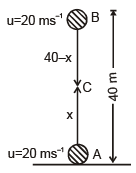40 – x = 20 × t + 1/2  × 9.8 t2
= 20t + 4.9 t2 ... (ii)
Adding (i) and (ii), 40 = 40 t   or t = 1 s
From (i), x = 20 × 1 – 4.9 × (1)2 = 15.1 m
Hence the two balls will collide after 1 s at a height of 15.1 m from the ground.

Offer running on EduRev: Apply code STAYHOME200 to get INR 200 off on our premium plan EduRev Infinity!

## Physics For JEE

188 videos|458 docs|276 tests

,

,

,

,

,

,

,

,

,

,

,

,

,

,

,

,

,

,

,

,

,

;